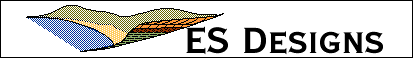working through the web...

### frontierMath

The frontierMath extension provides a cross-platform set of math functions to Frontier users. It provides the same functionality of the trigCMD and simpleMath UCMDs, but as a dll that will work with Frontier on both Windows and Mac machines. With v 1.1b2, the dll has been carbonized to work with Mac OS X. Many thanks to Andre Radke for the update.

The frontierMath extension provides the following basic math functions:

abs(x), acos(x), asin(x), atan(x), ceil(x), cos(x), cosh(x), exp(x), floor(x), log(x), log10, mod(x, y), pi(), power(x, y), round(x), roundToPlaces(x, n), sign(x), sin(x), sinh(x), sqrt(x), tan(x), tanh(x), trunc(x).

• conversion to and from scientific notation;
• numeric functions on lists of numbers;
• linear regression on lists of data; and
• functions to calculate statistical parameters.

The frontierMath extension was written for ES Designs by Arnold Lesikar, the developer of the simpleMath UCMD. The frontierMath extension will run on both Windows and MacPPC computers (Mac68K computers should continue to use the trigCMD and simpleMath UCMDs).